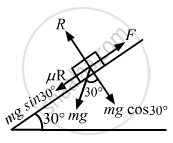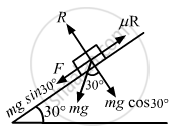Advertisement Remove all ads

# A Body of Mass 2 Kg is Lying on a Rough Inclined Plane of Inclination 30° - Physics

Sum

A body of mass 2 kg is lying on a rough inclined plane of inclination 30°. Find the magnitude of the force parallel to the incline needed to make the block move (a) up the incline (b) down the incline. Coefficient of static friction = 0.2.

Advertisement Remove all ads

#### Solution

(a) To make the block move up the incline, the applied force should be equal and opposite to the net force acting down the incline.
Applied force = μR + 2g sin 30°          (1)
(where μ is the coefficient of static friction)
R = mg cos 30°
Substituting the respective values in Equation (1), we get
=0.2xx(9.8)sqrt3+2xx9.8xx(1/2)
3.39 + 9.8 ≈ 13 NWith this minimum force, the body moves up the incline with a constant velocity as the net force on it is zero.
(b) Net force acting down the incline is given by
F = 2g sin 30° − μR=2xx9.8xx1/2-3.99
= 6.41 N
Because F = 6.41 N, the body will move down the incline with acceleration, hence the force required is zero.

Is there an error in this question or solution?
Advertisement Remove all ads

#### APPEARS IN

HC Verma Class 11, 12 Concepts of Physics 1
Chapter 6 Friction
Exercise | Q 6 | Page 97
Advertisement Remove all ads
Advertisement Remove all ads
Share
Notifications

View all notifications

Forgot password?